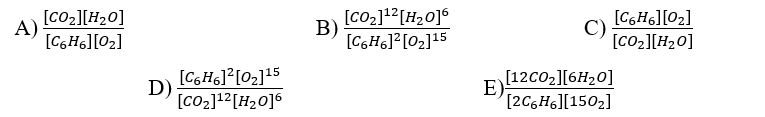# Problem: Write the equilibrium expression, Kc, for the following chemical reaction equation.2 C6H6(g) + 15 O2(g) ⇋ 12 CO2(g) + 6 H2O(g)

###### FREE Expert Solution
95% (314 ratings)
###### Problem Details

Write the equilibrium expression, Kc, for the following chemical reaction equation.

2 C6H6(g) + 15 O2(g) ⇋ 12 CO2(g) + 6 H2O(g)What scientific concept do you need to know in order to solve this problem?

Our tutors have indicated that to solve this problem you will need to apply the Equilibrium Expressions concept. If you need more Equilibrium Expressions practice, you can also practice Equilibrium Expressions practice problems.

What is the difficulty of this problem?

Our tutors rated the difficulty ofWrite the equilibrium expression, Kc, for the following chem...as medium difficulty.

How long does this problem take to solve?

Our expert Chemistry tutor, Dasha took 2 minutes and 42 seconds to solve this problem. You can follow their steps in the video explanation above.

What professor is this problem relevant for?

Based on our data, we think this problem is relevant for Professor Gill's class at UH.